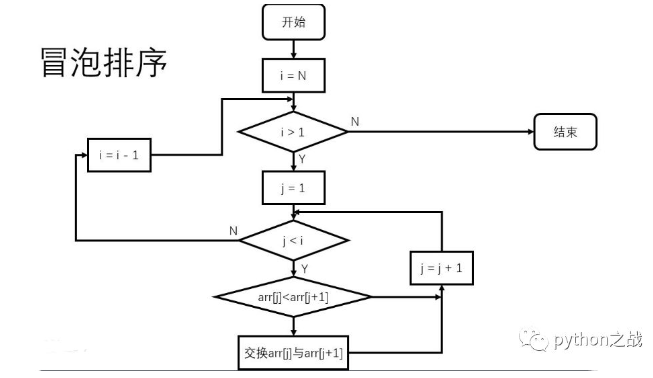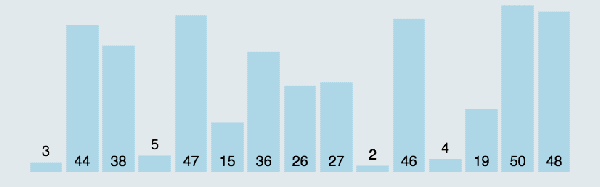# python实现冒泡排序算法python实现冒泡排序：

def bubble_sort(nums):

for i in range(len(nums) - 1):

for j in range(len(nums) - i - 1):

if nums[j] > nums[j + 1]:

nums[j], nums[j + 1] = nums[j + 1], nums[j]

return nums


python实现冒泡排序的核心思想是通过从列表一端迭代循环元素，再通过一个循环让这个元素之后的元素相邻两个比较，从而依次将最大值移动到最末端，如下图示意。